# Go Math Grade 4 Answer Key Homework Practice FL Chapter 8 Multiply Fractions by Whole Numbers

Students to master mathematical concepts must require the best resources to understand the logic and learn the concept easily. The only resource that helps all grade 4 students are Go Math Grade 4 Answer Key Homework Practice FL Chapter 8 Multiply Fractions by Whole Numbers. With the help of Grade 4 Go Math Solutions Homework Practice FL Ch 8 Multiply Fractions by Whole Numbers, students can learn the fundamentals and become a master in maths. So, students are recommended to utilize the detailed solutions covered in Go Math Grade 4 Answer Key Chapter 8.

## Go Math Grade 4 Answer Key Homework Practice FL Chapter 8 Multiply Fractions by Whole Numbers

Refer to the concepts of Homework Practice FL Chapter 8 Multiply Fractions by Whole Numbers before you ace up your preparation. Click on the links provided here and get a free pdf of chapterwise Go Math 4th Grade Answer Key Homework Practice FL Chapter 8 Multiply Fractions by Whole Numbers and practice the problems regularly on your own for experiencing the best learnings.

Lesson: 1 – Multiples of Unit Fractions

Lesson: 2 – Multiples of Fractions

Lesson: 3 – Multiply a Fraction by a Whole Number Using Models

Lesson: 4 – Multiply a Fraction or Mixed Number by a Whole Number.

Lesson: 5 – Problem Solving Comparison

Lesson: 6

### Common Core – Multiply Fractions by Whole Numbers – Page No. 157

Multiples of Unit Fractions

Write the fraction as a product of a whole number and a unit fraction.

Question 1.Explanation:
Given that 5/6 or 5 sixth-size parts.
Each sixth-size part of the given fraction can be shown by the unit fraction 1/6.
You can use unit fractions to show 5/6
5/6 = 5 x 1/6.

Question 2.
$$\frac{7}{8}$$
Type below:
_________

7 x 1/8

Explanation:
Given that 7/8 or 7 eighth-size parts.
Each eighth-size part of the given fraction can be shown by the unit fraction 1/8.
You can use unit fractions to show 7/8
7/8 = 7 x 1/8.

Question 3.
$$\frac{5}{3}$$
Type below:
_________

5 x 1/3

Explanation:
Given that 5/3 or 5 third-size parts.
Each third-size part of the given fraction can be shown by the unit fraction 1/3.
You can use unit fractions to show 5/6
5/3 = 5 x 1/3.

Question 4.
$$\frac{9}{10}$$
Type below:
_________

9 x 1/10

Explanation:
Given that 9/10 or 9 tenth-size parts.
Each tenth-size part of the given fraction can be shown by the unit fraction 1/10.
You can use unit fractions to show 9/10
9/10 = 9 x 1/10.

Question 5.
$$\frac{3}{4}$$
Type below:
_________

3 x 1/4

Explanation:
Given that 3/4 or 3 fourth-size parts.
Each fourth-size part of the given fraction can be shown by the unit fraction 1/4.
You can use unit fractions to show 5/6
3/4 = 3 x 1/4.

Question 6.
$$\frac{11}{12}$$
Type below:
_________

11 x 1/12

Explanation:
Given that 11/12 or 11 twelve-size parts.
Each twelve-size part of the given fraction can be shown by the unit fraction 1/12.
You can use unit fractions to show 5/6
11/12 = 11 x 1/12.

Question 7.
$$\frac{4}{6}$$
Type below:
_________

4 x 1/6

Explanation:
Given that 4/6 or 4 sixth-size parts.
Each sixth-size part of the given fraction can be shown by the unit fraction 1/6.
You can use unit fractions to show 4/6
4/6 = 4 x 1/6.

Question 8.
$$\frac{8}{20}$$
Type below:
_________

8 x 1/20

Explanation:
Given that 8/20 or 8 twenty-size parts.
Each twenty-size part of the given fraction can be shown by the unit fraction 1/20.
You can use unit fractions to show 8/20
8/20 = 8 x 1/20.

Question 9.
$$\frac{13}{100}$$
Type below:
_________

13 x 1/100

Explanation:
Given that 13/100 or 13 hundred-size parts.
Each hundred-size part of the given fraction can be shown by the unit fraction 1/100.
You can use unit fractions to show 13/100
13/100 = 13 x 1/100.

List the next four multiples of the unit fraction.

Question 10.
$$\frac{1}{5}$$,
Type below:
_________

2/5, 3/5, 4/5, 5/5

Explanation: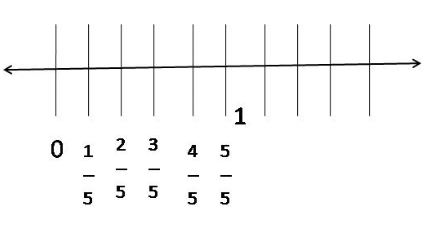2/5, 3/5, 4/5, 5/5

Question 11.
$$\frac{1}{8}$$,
Type below:
_________

2/8, 3/8, 4/8, 5/8

Explanation: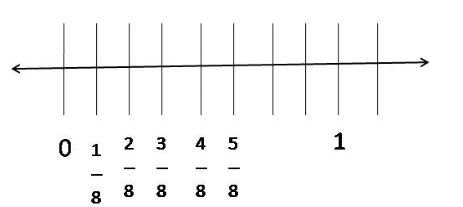2/8, 3/8, 4/8, 5/8

Problem Solving

Question 12.
So far, Monica has read $$\frac{5}{6}$$ of a book. She has read the same number of pages each day for 5 days. What fraction of the book does Monica read each day?
$$\frac{□}{□}$$ of the book

Explanation:
Monica has read 5/6 of a book. She has read the same number of pages each day for 5 days.
For 1 day, she read one page. In total, she read 5 pages in 5 days. So, Monica read 1/6 of a book each day.

Question 13.
So far, Monica has read $$\frac{3}{8}$$ of a book. She has read the same number of pages each day for 5 days. What fraction of the book does Monica read each day?
$$\frac{□}{□}$$ pound of cheese

Explanation:
Nicholas buys 3/8 pound of cheese. He bought 3 sandwiches. Then, he applied 3/8 pound of cheese on 3 sandwiches. So, 3 x 1/8 cheese he put on 3 sandwiches. So, for one sandwich he put 1/8 pound of cheese.

### Common Core – Multiply Fractions by Whole Numbers – Page No. 158

Lesson Check

Question 1.
Selena walks from home to school each morning and back home each afternoon. Altogether, she walks $$\frac{2}{3}$$ mile each day. How far does Selena live from school?
Options:
a. $$\frac{1}{3}$$ mile
b. $$\frac{2}{3}$$ mile
c. 1 $$\frac{1}{3}$$ miles
d. 2 miles

Explanation:
Selena walks from home to school each morning and back home each afternoon.
Altogether, she walks 2/3 miles each day.
The distance between home and school will remain the same.
So, 2/3 x 1/2 = 1/3 mile far Selena live from the school.
Thus the correct answer is option a.

Question 2.
Will uses $$\frac{3}{4}$$ cup of olive oil to make 3 batches of salad dressing. How much oil does Will use for one batch of salad dressing?
Options:
a. $$\frac{1}{4}$$ cup
b. $$\frac{1}{3}$$ cup
c. 2 $$\frac{1}{3}$$ cups
d. 3 cups

Answer: $$\frac{1}{4}$$ cup

Explanation:
Will uses 3/4 cups of olive oil to make 3 batches of salad dressing.
To know the one batch of salad dressing, we need to take one part of salad dressing = 1/3.
So, 3/4 x 1/3 = 1/4 cup of olive oil will use for one batch of salad dressing.
Thus the correct answer is option a.

Spiral Review

Question 3.
Liza bought $$\frac{5}{8}$$ pound of trail mix. She gives $$\frac{2}{8}$$ pound of trail mix to Michael. How much trail mix does Liza have left?
Options:
a. $$\frac{1}{8}$$ pound
b. $$\frac{2}{8}$$ pound
c. $$\frac{3}{8}$$ pound
d. $$\frac{4}{8}$$ pound

Explanation:
Liza bought 58 pound of trail mix. She gives 28 pounds of trail mix to Michael.
So, Liza has left 5/8 – 2/8 = 3/8 trail mix.
Thus the correct answer is option c.

Question 4.
Leigh has a piece of rope that is 6 $$\frac{2}{3}$$ feet long. How do you write 6 $$\frac{2}{3}$$ as a fraction greater than 1?
Options:
a. $$\frac{11}{3}$$ pound
b. $$\frac{15}{3}$$ pound
c. $$\frac{20}{3}$$ pound
d. $$\frac{62}{3}$$ pound

Explanation:
Multiply the denominator with the whole number. i.e Multiply 3 with 6 in the given example, 6 (2/3).
3 x 6 =18.
Keep the Denominator the same i.e. 3.
The obtained fraction is 20/3.
Thus the correct answer is option c.

Question 5.
Randy’s house number is a composite number. Which of the following could be Randy’s house number?
Options:
a. 29
b. 39
c. 59
d. 79

Explanation:
The composite numbers can be defined as the whole numbers that have more than two factors. Whole numbers that are not prime are composite numbers because they are divisible by more than two numbers. 39 is the composite number. 39 is divide by 13 and 3.
Thus the correct answer is option b.

Question 6.
Mindy buys 12 cupcakes. Nine of the cupcakes have chocolate frosting and the rest have vanilla frosting. What fraction of the cupcakes have vanilla frosting?
Options:
a. $$\frac{1}{4}$$
b. $$\frac{1}{3}$$
c. $$\frac{2}{3}$$
d. $$\frac{3}{4}$$

Explanation:
Nine of the cupcakes have chocolate frosting = 9/12.
The rest have vanilla frosting. So, there are 3 cups remained = 3/12 = 1/4.
1/4 cupcakes have vanilla frosting.
Thus the correct answer is option a.

### Common Core – Multiply Fractions by Whole Numbers – Page No. 159

Multiples of Fractions

List the next four multiples of the fraction.

Question 1.
$$\frac{3}{5}$$,
Type below:
_________

6/5, 9/5, 12/5, 20/5

Explanation:
1 x 3/5 = 3/5.
2 x 3/5 = 6/5.
3 x 3/5 = 9/5.
4 x 3/5 = 12/5.
5 x 4/5 = 20/5.
The next four multiples of 3/5 are 6/5, 9/5, 12/5, 20/5.

Question 2.
$$\frac{2}{6}$$,
Type below:
_________

4/6, 6/6, 8/6, 10/6

Explanation:
1 x 2/6 = 2/6.
2 x 2/6 = 4/6.
3 x 2/6 = 6/6.
4 x 2/6 = 8/6.
5 x 2/6 = 10/6.
The next four multiples of 2/6 are 4/6, 6/6, 8/6, 10/6.

Question 3.
$$\frac{4}{8}$$,
Type below:
_________

8/8, 12/8, 16/8, 20/8

Explanation:
1 x 4/8 = 4/8.
2 x 4/8 = 8/8.
3 x 4/8 = 12/8.
4 x 4/8 = 16/8.
5 x 4/8 = 20/8.
The next four multiples of 4/8 are 8/8, 12/8, 16/8, 20/8.

Question 4.
$$\frac{5}{10}$$,
Type below:
_________

10/10, 15/10, 20/10, 25/10

Explanation:
1 x 5/10 = 5/10.
2 x 5/10 = 10/10.
3 x 5/10 = 15/10.
4 x 5/10 = 20/10.
5 x 5/10 = 25/10.
The next four multiples of 5/10 are 10/10, 15/10, 20/10, 25/10.

Write the product as the product of a whole number and a unit fraction.

Question 5.2 × $$\frac{4}{5}$$ =
Type Below:
_________

Answer: 8/5 = 8 x 1/5

Explanation:
1 group of 4/5 = 4/5
2 groups of 4/5 = 8/5
2 x 4/5 = 8/5 = 8 x 1/5.

Question 6.5 × $$\frac{2}{3}$$ =
Type below:
_________

10/3 = 10 x 1/3

Explanation:
1 group of 2/3 = 2/3
2 group of 2/3 = 4/3
3 group of 2/3 = 6/3
4 group of 2/3 = 8/3
5 group of 2/3 = 10/3
5 x 2/3 = 10/3 = 10 x 1/3.

Problem Solving

Question 7.
Jessica is making 2 loaves of banana bread. She needs $$\frac{3}{4}$$ cup of sugar for each loaf. Her measuring cup can only hold $$\frac{1}{4}$$ cup of sugar. How many times will Jessica need to fill the measuring cup in order to get enough sugar for both loaves of bread?
_____ times

Explanation:
Jessica is making 2 loaves of banana bread. She needs a 3/4 cup of sugar for each loaf.
For 2 loaves, she needs 2 x 3/4 = 6/4 cups of sugar.
Her measuring cup can only hold 1/4 cup of sugar. So, to get the 3/4 cup of sugar, she needs to fill the cup 3 times. 1/4 + 1/4 + 1/4 = 3/4.
So, to fill 2 loaves, she needs to fill cup 3 x 2 = 6 times.

Question 8.
A group of four students is performing an experiment with salt. Each student must add $$\frac{3}{8}$$ teaspoon of salt to a solution. The group only has a $$\frac{1}{8}$$ teaspoon measuring spoon. How many times will the group need to fill the measuring spoon in order to perform the experiment?
_____ times

Explanation:
A group of four students is performing an experiment with salt. Each student must add a 3/8 teaspoon of salt to a solution. 4 x 3/8 = 12/8 teaspoon of salt required to finish the experiment.
If they have 1/8 teaspoon measuring spoon, 12 x 1/8.
So, the group needs to fill the measuring spoon 12 times in order to perform the experiment.

### Common Core – Multiply Fractions by Whole Numbers – Page No. 160

Lesson Check

Question 1.
Eloise made a list of some multiples of $$\frac{5}{8}$$. Which of the following lists could be Eloise’s list?
Options:
a. $$\frac{5}{8}, \frac{10}{16}, \frac{15}{24}, \frac{20}{32}, \frac{25}{40}$$
b. $$\frac{5}{8}, \frac{10}{8}, \frac{15}{8}, \frac{20}{8}, \frac{25}{8}$$
c. $$\frac{5}{8}, \frac{6}{8}, \frac{7}{8}, \frac{8}{8}, \frac{9}{8}$$
d. $$\frac{1}{8}, \frac{2}{8}, \frac{3}{8}, \frac{4}{8}, \frac{5}{8}$$

Answer: b. 5/8, 10/8, 15/8, 20/8, 25/8
Explanation:
1 x 5/8 = 5/8.
2 x 5/8 = 10/8.
3 x 5/8 = 15/8.
4 x 5/8 = 20/8.
5 x 5/8 = 25/8.
The next four multiples of 5/8 are $$\frac{5}{8}, \frac{10}{8}, \frac{15}{8}, \frac{20}{8}, \frac{25}{8}$$
Thus the correct answer is option b.

Question 2.
David is filling five $$\frac{3}{4}$$ quart bottles with a sports drink. His measuring cup only holds $$\frac{1}{4}$$ quart. How many times will David need to fill the measuring cup in order to fill the 5 bottles?
Options:
a. 5
b. 10
c. 15
d. 20

Explanation:
David is filling five 3/4 quart bottles with a sports drink = 5 x 3/4 = 15/4.
His measuring cup only holds 1/4 quart.
So, 15 x 1/4. David needs to fill the measuring cup 15 times in order to fill the 5 bottles.
Thus the correct answer is option c.

Spiral Review

Question 3.
Ira has 128 stamps in his stamp album. He has the same number of stamps on each of the 8 pages. How many stamps are on each page?
Options:
a. 12
b. 14
c. 16
d. 18

Explanation:
Ira has 128 stamps in his stamp album. He has the same number of stamps on each of the 8 pages.
128/8 = 16 stamps on each page.
So, there are 16 stamps on each page.
Thus the correct answer is option b.

Question 4.
Ryan is saving up for a bike that costs $198. So far, he has saved$15 per week for the last 12 weeks. How much more money does Ryan need in order to be able to buy the bike?
Options:
a. $8 b.$ 18
c. $48 d.$ 180

Answer: b. $18 Explanation: Ryan is saving up for a bike that costs$198.
So far, he has saved $15 per week for the last 12 weeks =$15 x 12 = $180.$198 – $180 =$18 need in order to buy the bike.
Thus the correct answer is option b.

Question 5.
Tina buys 3 $$\frac{7}{8}$$ yards of material at the fabric store. She uses it to make a skirt. Afterward, she has 1 $$\frac{3}{8}$$ yards of the fabric leftover. How many yards of material did Tina use?
Options:
a. 1 $$\frac{4}{8}$$
b. 2 $$\frac{1}{8}$$
c. 2 $$\frac{4}{8}$$
d. 5 $$\frac{2}{8}$$

Explanation:
Tina buys 3 7/8 yards of material at the fabric store. She uses it to make a skirt. Afterward, she has 1 3/8 yards of the fabric leftover.
3 -1 = 2; 7/8 – 3/8 = 4/8.
So, the answer is 2 $$\frac{4}{8}$$.
Thus the correct answer is option c.

Question 6.
Which list shows the fractions in order from least to greatest?
Options:
a. $$\frac{2}{3}, \frac{3}{4}, \frac{7}{12}$$
b. $$\frac{7}{12}, \frac{3}{4}, \frac{2}{3}$$
c. $$\frac{3}{4}, \frac{2}{3}, \frac{7}{12}$$
d. $$\frac{7}{12}, \frac{2}{3}, \frac{3}{4}$$

Answer: d. $$\frac{7}{12}, \frac{2}{3}, \frac{3}{4}$$

Explanation:
2/3 = 0.666
3/4 = 0.75
7/12 = 0.5833
$$\frac{7}{12}, \frac{2}{3}, \frac{3}{4}$$
Thus the correct answer is option d.

### Common Core – Multiply Fractions by Whole Numbers – Page No. 161

Multiply a Fraction by a Whole Number Using Models

Multiply.

Question 1.Question 2.
3 × $$\frac{2}{5}$$ = $$\frac{□}{□}$$3 x 2/5 = 6/5

Question 3.
7 × $$\frac{3}{10}$$ = $$\frac{□}{□}$$7 x 3/10 = 21/10

Question 4.
3 × $$\frac{5}{12}$$ = $$\frac{□}{□}$$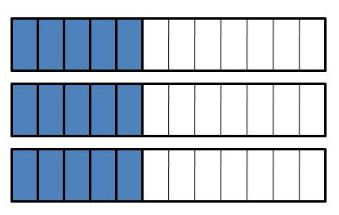3 x 5/12 = 15/12

Question 5.
6 × $$\frac{3}{4}$$ = $$\frac{□}{□}$$6 x 3/4 = 18/4

Question 6.
4 × $$\frac{2}{8}$$ = $$\frac{□}{□}$$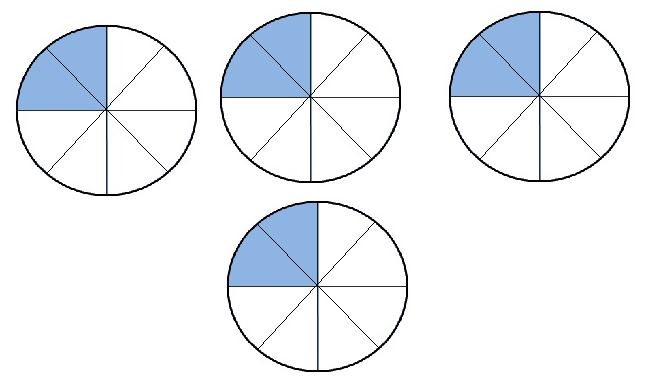4 x 2/8 = 8/8

Question 7.
5 × $$\frac{2}{3}$$ = $$\frac{□}{□}$$5 x 2/3 = 10/3

Question 8.
2 × $$\frac{7}{8}$$ = $$\frac{□}{□}$$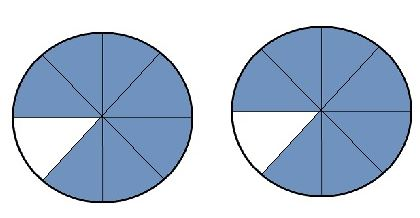2 x 7/8 = 14/8

Question 9.
6 × $$\frac{4}{5}$$ = $$\frac{□}{□}$$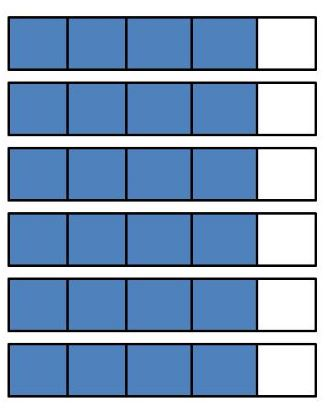6 x 4/5 = 28/5

Problem Solving

Question 10.
Matthew walks $$\frac{5}{8}$$ mile to the bus stop each morning. How far will he walk in 5 days?
$$\frac{□}{□}$$

Explanation:
Matthew walks 5/8 mile to the bus stop each morning.
In 5 days, 5 x 5/8 = 25/8 miles.

Question 11.
Emily uses $$\frac{2}{3}$$ cup of milk to make one batch of muffins. How many cups of milk will Emily use if she makes 3 batches of muffins?
$$\frac{□}{□}$$

Explanation:
Emily uses a 2/3 cup of milk to make one batch of muffins.
Emily use 3 x 2/3 = 6/3 cups of milk to make 3 batches of muffins

### Common Core – Multiply Fractions by Whole Numbers – Page No. 162

Lesson Check

Question 1.
Aleta’s puppy gained $$\frac{3}{8}$$ pound each week for 4 weeks. Altogether, how much weight did the puppy gain during the 4 weeks?
Options:
a. $$\frac{8}{12}$$ pound
b. 1 $$\frac{2}{8}$$ pounds
c. $$\frac{12}{8}$$ pounds
d. 4 $$\frac{3}{8}$$ pounds

Answer: $$\frac{12}{8}$$ pounds

Explanation:
Aleta’s puppy gained 3/8 pound each week.
It gained 4 x 3/8 = 12/8 pounds in 4 weeks.
Thus the correct answer is option c.

Question 2.
Pedro mixes $$\frac{3}{4}$$ teaspoon of plant food into each gallon of water. How many teaspoons of plant food should Pedro mix into 5 gallons of water?
Options:
a. $$\frac{3}{20}$$ teaspoon
b. $$\frac{4}{15}$$ teaspoon
c. $$\frac{8}{4}$$ teaspoons
d. $$\frac{15}{4}$$ teaspoons

Answer: d. $$\frac{15}{4}$$ teaspoons

Explanation:
If Pedro mixes 3/4 teaspoon of plant food into each gallon of water, then 5 x 3/4 = 15/4 teaspoon of plant food mix into 5 gallons of water.
Thus the correct answer is option d.

Spiral Review

Question 3.
Ivana has $$\frac{3}{4}$$pound of hamburger meat. She makes 3 hamburger patties. Each patty weighs the same amount. How much does each hamburger patty weigh?
Options:
a. $$\frac{1}{4}$$ pound
b. $$\frac{1}{3}$$ pound
c. 2 $$\frac{1}{4}$$ pounds
d. 3 pounds

Answer: a. $$\frac{1}{4}$$ pound

Explanation:
Ivana has 3/4 pound of hamburger meat. She makes 3 hamburger patties.
Each patty weighs the same amount. So, each hamburger patty weighs 1/4 pound.
Thus the correct answer is option a.

Question 4.
Which of the following expressions is NOT equal to $$\frac{7}{10}$$?
Options:
a. $$\frac{5}{10}+\frac{1}{10}+\frac{1}{10}$$
b. $$\frac{2}{10}+\frac{2}{10}+\frac{3}{10}$$
c. $$\frac{3}{10}+\frac{3}{10}+\frac{2}{10}$$
d. $$\frac{4}{10}+\frac{2}{10}+\frac{1}{10}$$

Explanation:
a. 5/10+1/10+1/10 = 7/10
b. 2/10+2/10+3/10 = 7/10
c. 3/10+3/10+2/10 = 8/10
d. 4/10+2/10+1/10 = 7/10
The expression not equal to $$\frac{7}{10}$$ is $$\frac{8}{10}$$
Thus the correct answer is option c.

Question 5.
Lance wants to find the total length of 3 boards. He uses the expression $$3 \frac{1}{2}+\left(2+4 \frac{1}{2}\right)$$. How can Lance rewrite the expression using both the Associative and Commutative Properties of Addition?
Options:
a. $$5+4 \frac{1}{2}$$
b. $$\left(3 \frac{1}{2}+2\right)+4 \frac{1}{2}$$
c. $$2+\left(3 \frac{1}{2}+4 \frac{1}{2}\right)$$
d. $$3 \frac{1}{2}+\left(4 \frac{1}{2}+2\right)$$

Answer: She can write as (3 1/2 + 2) + 4 1/2

Question 6.
Which of the following statements is true?
Options:
a. $$\frac{5}{8}>\frac{9}{10}$$
b. $$\frac{5}{12}>\frac{1}{3}$$
c. $$\frac{3}{6}>\frac{4}{5}$$
d. $$\frac{1}{2}>\frac{3}{4}$$

Answer: $$\frac{1}{2}>\frac{3}{4}$$

Explanation:
0.625 > 0.9
0.416 > 0.333
0.5 > 0.8
0.5 > 0.75
Thus the correct answer is option d.

### Common Core – Multiply Fractions by Whole Numbers – Page No. 163

Multiply a Fraction or Mixed Number by a Whole Number.

Multiply. Write the product as a mixed number.

Question 1.1  5/10

Explanation:
5 × 3/10 = 15/10 = 1 and remainder is 5. So, the mixed fraction is 1  5/10

Question 2.
3 × $$\frac{3}{5}$$ =
_____ $$\frac{□}{□}$$

1 × 4/5

Explanation:
3 × 3/5 = 9/5 = 1 and remainder is 4. So, the mixed fraction is 1  4/5

Question 3.
5 × $$\frac{3}{4}$$ =
_____ $$\frac{□}{□}$$

3  3/4
Explanation:
15/4 = 3 and the remainder is 3. So, the mixed fraction is 3  3/4

Question 4.
4 × 1 $$\frac{1}{5}$$ =
_____ $$\frac{□}{□}$$

4  4/5
Explanation:
1 ×15 = 6/5.
4 x 6/5 = 24/5 = 4 and the remainder is 4. So, the mixed fraction is 4× 4/5

Question 5.
2 × 2 $$\frac{1}{3}$$ =
_____ $$\frac{□}{□}$$

4  2/3
Explanation:
2 13 = 7/3.
2 x 7/3 = 14/3.
14/3 = 4 and the remainder is 2. So, the mixed fraction is 4 2/3

Question 6.
5 × 1 $$\frac{1}{6}$$ =
_____ $$\frac{□}{□}$$

Explanation:
1 1/6 = 7/6
5 x 7/6 = 35/6.
35/6 = 5 and the remainder is 5.
So, the mixed fraction is 5 5/6

Question 7.
2 × 2 $$\frac{7}{8}$$ =
_____ $$\frac{□}{□}$$

Explanation:
2 7/8 = 23/8
2 x 23/8 = 46/8 = 6 1/1

Question 8.
7 × 1 $$\frac{3}{4}$$ =
_____ $$\frac{□}{□}$$

Explanation:
1 3/4 = 7/4
7 x 7/4 = 39/4
39/4 = 9 and the remainder is 3.
So, the mixed fraction is 9 3/4

Question 9.
8 × 1 $$\frac{3}{5}$$ =
_____ $$\frac{□}{□}$$

Explanation:
1 3/5 = 8/5
8 x 8/5 = 64/5
64/5 = 12 and the remainder is 4.
So, the mixed fraction is 12 4/5

Problem Solving

Question 10.
Brielle exercises for $$\frac{3}{4}$$ hour each day for 6 days in a row. Altogether, how many hours does she exercise during the 6 days?
_____ $$\frac{□}{□}$$

Explanation:
6 x 3/4 = 18/4 = 4 and the remainder is 2.
So, the mixed fraction is 4 2/4.

Question 11.
A recipe for quinoa calls for 2 $$\frac{2}{3}$$ cups of milk. Conner wants to make 4 batches of quinoa. How much milk does he need?
_____ $$\frac{□}{□}$$

Explanation:
quinoa calls for 8/3 cups of milk. Conner wants to make 4 batches of quinoa.
So, 4 x 8/3 = 32/3 = 10 and the remainder is 2.
So, the mixed fraction is 10 2/3

### Common Core – Multiply Fractions by Whole Numbers – Page No. 164

Lesson Check

Question 1.
A mother is 1 $$\frac{3}{4}$$ times as tall as her son. Her son is 3 feet tall. How tall is the mother?
Options:
a. 4 $$\frac{3}{4}$$ feet
b. 5 $$\frac{1}{4}$$ feet
c. 5 $$\frac{1}{2}$$ feet
d. 5 $$\frac{3}{4}$$ feet

Explanation:
A mother is 1 3/4 times as tall as her son. Her son is 3 feet tall.
So, 3 x 7/4 = 21/4 = 5 and the remainder is 1.
The mixed fraction is 5 1/4 feet.
Thus the correct answer is option b.

Question 2.
The cheerleaders are making a banner that is 8 feet wide. The length of the banner is 1 $$\frac{1}{3}$$ times the width of the banner. How long is the banner?
Options:
a. 8 $$\frac{1}{3}$$ feet
b. 8 $$\frac{3}{8}$$ feet
c. 10 $$\frac{1}{3}$$ feet
d. 10 $$\frac{2}{3}$$ feet

Explanation:
The cheerleaders are making a banner that is 8 feet wide. The length of the banner is 1 1/3 times the width of the banner.
So, 8 x 4/3 = 32/3 =10 and the remainder is 2.
The mixed fraction is 10 2/3 feet.
Thus the correct answer is option d.

Spiral Review

Question 3.
Karleigh walks $$\frac{5}{8}$$ mile to school every day. How far does she walk to school in 5 days?
Options:
a. $$\frac{5}{40}$$ mile
b. $$\frac{25}{40}$$ mile
c. $$\frac{10}{8}$$ miles
d. $$\frac{25}{8}$$ miles

Explanation:
5 x 5/8 = 25/8.
Thus the correct answer is option d.

Question 4.
Which number is a multiple of $$\frac{4}{5}$$?
Options:
a. $$\frac{8}{10}$$
b. $$\frac{12}{15}$$
c. $$\frac{16}{20}$$
d. $$\frac{12}{5}$$

Explanation:
The multiple of 45 has the denominator 5.
So, 12/5 is the correct answer.
Thus the correct answer is option d.

Question 5.
Jo cut a key lime pie into 8 equal-size slices. The next day, $$\frac{7}{8}$$ of the pie is left. Jo puts each slice on its own plate. How many plates does she need?
Options:
a. 5
b. 6
c. 7
d. 8

Explanation:
Jo cut a key lime pie into 8 equal-size slices.
The next day, 78 of the pie is left. Jo puts each slice on its own plate.
She needs 7 plates.
Thus the correct answer is option c.

Question 6.
Over the weekend, Ed spent 1 $$\frac{1}{4}$$ hours doing his math homework and 1 $$\frac{3}{4}$$ hours doing his science project. Altogether, how much time did Ed spend doing homework over the weekend?
Options:
a. 3 hours
b. 2 $$\frac{3}{4}$$ hours
c. 2 $$\frac{1}{2}$$ hours
d. 2 hours

Explanation:
Given,
Over the weekend, Ed spent 1 $$\frac{1}{4}$$ hours doing his math homework and 1 $$\frac{3}{4}$$ hours doing his science project.
5/4 + 7/4 = 12/4 = 3 hours
Thus the correct answer is option a.

### Common Core – Multiply Fractions by Whole Numbers – Page No. 165

Problem Solving Comparison

Problems with Fractions

Question 1.
A shrub is 1 $$\frac{2}{3}$$ feet tall. A small tree is 3 times as tall as the shrub. How tall is the tree?Explanation:Question 2.
You run 1 $$\frac{3}{4}$$ miles each day. Your friend runs 4 times as far as you do. How far does your friend run each day?
_________ miles

Explanation:
Given,
You run 1 $$\frac{3}{4}$$ miles each day.
Your friend runs 4 times as far as you do.
4 x 7/4 = 7 miles each day

Question 3.
At the grocery store, Ayla buys 1 $$\frac{1}{3}$$ pounds of ground turkey. Tasha buys 2 times as much ground turkey as Ayla. How much ground turkey does Tasha buy?
_____ $$\frac{□}{□}$$ pounds

Explanation:
Given,
At the grocery store, Ayla buys 1 $$\frac{1}{3}$$ pounds of ground turkey.
Tasha buys 2 times as much ground turkey as Ayla.
2 x 4/3 = 8/3 = 2 and the remainder is 2.
The mixed fraction is 2 2/3 pounds.

Question 4.
When Nathan’s mother drives him to school, it takes $$\frac{1}{5}$$ hour. When Nathan walks to school, it takes him 4 times as long to get to school. How long does it take Nathan to walk to school?
$$\frac{□}{□}$$ hours

Explanation:
Given,
When Nathan’s mother drives him to school, it takes $$\frac{1}{5}$$ hour.
When Nathan walks to school, it takes him 4 times as long to get to school.
4 x 1/5 = 4/5 hour
It takes 4/5 hour Nathan to walk to school.

### Common Core – Multiply Fractions by Whole Numbers – Page No. 166

Lesson Check

Question 1.
A Wilson’s Storm Petrel is a small bird with a wingspan of 1 $$\frac{1}{3}$$ feet. A California Condor is a larger bird with a wingspan almost 7 times as wide as the wingspan of the petrel. About how wide is the wingspan of the California Condor?
Options:
a. $$\frac{4}{21}$$ foot
b. 2 $$\frac{1}{3}$$ feet
c. 7 $$\frac{1}{3}$$ feet
d. 9 $$\frac{1}{3}$$ feet

Explanation:
Given,
A Wilson’s Storm Petrel is a small bird with a wingspan of 1 $$\frac{1}{3}$$ feet.
A California Condor is a larger bird with a wingspan almost 7 times as wide as the wingspan of the petrel.
Convert from mixed fraction to the improper fraction.
1 1/3 = 4/3.
7 x 4/3 = 28/3 feet = 9 and the remainder is 1.
The mixed fraction is 9 1/3
Thus the correct answer is option d.

Question 2.
The walking distance from the Empire State Building in New York City to Times Square is about $$\frac{9}{10}$$ mile. The walking distance from the Empire State Building to Sue’s hotel is about 8 times as far. About how far is Sue’s hotel from the Empire State Building?
Options:
a. $$\frac{9}{80}$$ mile
b. $$\frac{72}{80}$$ mile
c. 1 $$\frac{7}{10}$$ miles
d. 7 $$\frac{2}{10}$$ miles

Explanation:
Given,
The walking distance from the Empire State Building in New York City to Times Square is about $$\frac{9}{10}$$ mile.
The walking distance from the Empire State Building to Sue’s hotel is about 8 times as far.
8 x 9/10 mile = 72/10 mile = 7 and the remainder is 2.
The mixed fraction is 7 2/10 miles.
Thus the correct answer is option d.

Spiral Review

Question 3.
Which of the following expressions is NOT equal to 3 × 2 $$\frac{1}{4}$$?
Options:
a. $$3 \times \frac{9}{4}$$
b. (3 × 2) + (3 × $$\frac{1}{4}$$)
c. 6 $$\frac{3}{4}$$
d. 3 × 2 + $$\frac{1}{4}$$

Answer: d. 3 × 2 + 14

Explanation:
3 × 2 14 = 3 x 9/4 = 27/4
a. 3 × 94 = 27/4
b. (3 × 2) + (3 × 14) = 6 + 3/4 = 27/4
c. 6 3/4 = 27/4
d. 3 × 2 + 14 = 6 + 1/4 = 25/4
Thus the correct answer is option d.

Question 4.
At a bake sale, Ron sells $$\frac{7}{8}$$ of an apple pie and $$\frac{5}{8}$$ of a cherry pie. Altogether, how much pie does he sell at the bake sale?
Options:
a. $$\frac{2}{8}$$
b. $$\frac{12}{16}$$
c. $$\frac{12}{8}$$
d. $$\frac{35}{8}$$

Explanation:
Given,
At a bake sale, Ron sells $$\frac{7}{8}$$ of an apple pie and $$\frac{5}{8}$$ of a cherry pie.
7/8 + 5/8 = 12/8
The bake sale 12/8 pie.
Thus the correct answer is option c.

Question 5.
On a ruler, which measurement is between $$\frac{3}{16}$$ inch and $$\frac{7}{8}$$ inch?
Options:
a. $$\frac{1}{16}$$ inch
b. $$\frac{1}{8}$$ inch
c. $$\frac{11}{16}$$ inch
d. $$\frac{15}{16}$$ inch

Explanation:
Subtract $$\frac{3}{16}$$ inch and $$\frac{7}{8}$$
Make denominators as common.
$$\frac{7}{8}$$ × $$\frac{2}{2}$$ = $$\frac{14}{16}$$
$$\frac{14}{16}$$ – $$\frac{3}{16}$$ = $$\frac{11}{16}$$ inch.
Thus the correct answer is option c.

Question 6.
Which of the following numbers is composite?
Options:
a. 4
b. 3
c. 2
d. 1

a. 4

Explanation:
A composite number is a positive integer that can be formed by multiplying two smaller positive integers. Equivalently, it is a positive integer that has at least one divisor other than 1 and itself.
The factors of 4 are 1, 2, 4.
4 has more than 2 factors.
Thus the correct answer is option a.

### Common Core – Multiply Fractions by Whole Numbers – Page No. 167

Lesson 8.1

Write the fraction as a product of a whole number and a unit fraction.

Question 1.
$$\frac{5}{6}$$ =
Type below:
________

Explanation:
Given that 5/6 or 5 sixth-size parts.
Each sixth-size part of the given fraction can be shown by the unit fraction 1/6.
You can use unit fractions to show 5/6
$$\frac{5}{6}$$ = 5 x 1/6.

Question 2.
$$\frac{7}{8}$$ =
Type below:
________

Explanation:
Given that 7/8 or 7 eighth-size parts.
Each eighth-size part of the given fraction can be shown by the unit fraction 1/8.
You can use unit fractions to show 7/8
$$\frac{7}{8}$$ = 7 x 1/8.

Question 3.
$$\frac{3}{5}$$ =
Type below:
________

Explanation:
Given that 5/3 or 5 third-size parts.
Each third-size part of the given fraction can be shown by the unit fraction 1/3.
You can use unit fractions to show 5/6
5/3 = 5 x 1/3.

List the next four multiples of the unit fraction

Question 4.
$$\frac{1}{2}$$,
Type below:
________

Explanation:
1 x 1/2 = 1/2.
2 x 1/2 = 2/2.
3 x 1/2 = 3/2.
4 x 1/2 = 4/2.
5 x 1/2 = 5/2.
The next four multiples of 1/2 are 2/2, 3/2, 4/2, 5/2.

Question 5.
$$\frac{1}{6}$$,
Type below:
________

Explanation:
1 x 1/6 = 1/6.
2 x 1/6 = 2/6.
3 x 1/6 = 3/6.
4 x 1/6 = 4/6.
5 x 1/6 = 5/6.
6 x 1/6 = 6/6.
The next four multiples of 1/6 are 2/6, 3/6, 4/6, 5/6,6/6.

Lesson 8.2

List the next four multiples of the fraction.

Question 6.
$$\frac{3}{10}$$,
Type below:
________

Explanation:
1 × 3/10 = 3/10
2 × 3/10 = 6/10
3 × 3/10 = 9/10
4 × 3/10 = 12/10
5 × 3/10 = 15/10

Question 7.
$$\frac{7}{12}$$,
Type below:
________

Answer: 7/12, 14/12, 21/12, 28/12, 35/12

Explanation:
1 × 7/12 = 7/12
2 × 7/12 = 14/12
3 × 7/12 = 21/12
4 × 7/12 = 28/12
5 × 7/12 = 35/12

Write the product as the product of a whole number and a unit fraction.

Question 8.2 × $$\frac{3}{6}$$ =
Type below:
________

1 group of $$\frac{3}{6}$$ is $$\frac{3}{6}$$
2 groups of $$\frac{3}{6}$$ is $$\frac{6}{6}$$
2 × $$\frac{3}{6}$$ = $$\frac{6}{6}$$

Question 9.3 × $$\frac{2}{8}$$ =
Type below:
________

Explanation:
1 group of $$\frac{2}{8}$$ is $$\frac{2}{8}$$
2 group of $$\frac{2}{8}$$ is $$\frac{4}{8}$$
3 group of $$\frac{2}{8}$$ is $$\frac{6}{8}$$
3 × $$\frac{2}{8}$$ = $$\frac{6}{8}$$

### Common Core – Multiply Fractions by Whole Numbers – Page No. 168

Lesson 8.3

Multiply.

Question 1.
3 × $$\frac{7}{10}$$ =
$$\frac{□}{□}$$

Answer: $$\frac{21}{10}$$

Explanation:
Multiply 7 and 3
3 × 7 = 21
3 × $$\frac{7}{10}$$ = $$\frac{21}{10}$$

Question 2.
5 × $$\frac{4}{8}$$ =
$$\frac{□}{□}$$

Explanation:
Multiply 5 and 4
5 × 4 = 20
5 × $$\frac{4}{8}$$ = $$\frac{20}{8}$$

Question 3.
4 × $$\frac{6}{12}$$ =
$$\frac{□}{□}$$

Explanation:
Multiply 4 and 6
4 × 6 = 24
4 × $$\frac{6}{12}$$ = $$\frac{24}{12}$$

Question 4.
2 × $$\frac{3}{4}$$ =
$$\frac{□}{□}$$

Explanation:
Multiply 2 and 3
2 × 3 = 6
2 × $$\frac{3}{4}$$ = $$\frac{6}{4}$$

Question 5.
6 × $$\frac{3}{5}$$ =
$$\frac{□}{□}$$

Explanation:
Multiply 6 and 3
6 × 3 =18
6 × $$\frac{3}{5}$$ = $$\frac{18}{5}$$

Question 6.
7 × $$\frac{2}{10}$$ =
$$\frac{□}{□}$$

Explanation:
Multiply 7 and 2.
7 × 2 =14
7 × $$\frac{2}{10}$$ = $$\frac{14}{10}$$

Lesson 8.4

Multiply. Write the product as a mixed number.

Question 7.
4 × $$\frac{8}{10}$$ =
_____ $$\frac{□}{□}$$

Explanation:
Given,
4 × $$\frac{8}{10}$$
First multiply 4 and 8
4 × 8 = 32
4 × $$\frac{8}{10}$$ = 32/10
Now convert from improper fraction to the mixed fraction.
32/10 = 3 $$\frac{2}{10}$$

Question 8.
3 × $$\frac{5}{6}$$ =
_____ $$\frac{□}{□}$$

Explanation:
Given,
3 × $$\frac{5}{6}$$
First multiply 3 and 5.
3 × 5 =15
3 × $$\frac{5}{6}$$ = 15/6
Now convert from improper fraction to the mixed fraction.
15/6 = 2 3/6

Question 9.
2 × 3 $$\frac{1}{3}$$ =
_____ $$\frac{□}{□}$$

Explanation:
Given,
2 × 3 $$\frac{1}{3}$$
3 $$\frac{1}{3}$$ = 10/3
2 × 10/3 = 20/3
Now convert from improper fraction to the mixed fraction.
20/3 = 6 2/3

Question 10.
4 × 2 $$\frac{2}{5}$$ =
_____ $$\frac{□}{□}$$

Explanation:
Given,
4 × 2 $$\frac{2}{5}$$
2 $$\frac{2}{5}$$ = 4/5
4 × 12/5 = 48/5
Now convert from improper fraction to the mixed fraction.
48/5 = 9 3/5

Question 11.
5 × 1 $$\frac{7}{8}$$ =
_____ $$\frac{□}{□}$$

Explanation:
Given,
5 × 1 $$\frac{7}{8}$$
5 × 15/5 = 75/5
Now convert from improper fraction to the mixed fraction.
75/5 = 9 3/8

Question 12.
3 × 3 $$\frac{3}{4}$$ =
_____ $$\frac{□}{□}$$

Explanation:
Given,
3 × 3 $$\frac{3}{4}$$
3 × 15/4 = 45/4
Now convert from improper fraction to the mixed fraction.
45/4 = 11 1/4

Lesson 8.5

Question 13.
A shrub in Pam’s back yard is about 1 $$\frac{3}{8}$$ feet tall. A small tree in her back yard is 7 times as tall as the shrub. About how tall is the tree?
_____ $$\frac{□}{□}$$ feet

Explanation:
Given,
A shrub in Pam’s back yard is about 1 $$\frac{3}{8}$$ feet tall.
A small tree in her back yard is 7 times as tall as the shrub.
9.625 ft because 1 3/8 × 7 is equal to 9 5/2 feet
Therefore the tree is 9 5/2 feet.

Question 14.
A puppy weighs $$\frac{9}{10}$$ pound. Its mother weighs 8 times as much. How much does the mother weigh?
_____ $$\frac{□}{□}$$ pounds

Answer: 7 $$\frac{2}{10}$$ pounds

Explanation:
Given,
A puppy weighs $$\frac{9}{10}$$ pound. Its mother weighs 8 times as much.
$$\frac{9}{10}$$ × 8 = 72/10
Convert from improper fraction to the mixed fraction.
72/10 = 7 $$\frac{2}{10}$$ pounds
Thus the mother weigh 7 $$\frac{2}{10}$$ pounds.

### Conclusion:

Here the provided HMH Go Math Solution Key for Grade 4 Homework Practice FL Chapter 8 Multiply Fractions by Whole Numbers will increase your subject knowledge & get good scores in the exams. By understanding the difficulty of fractions, it can be easy to grasp the logic and tricks to solve the covered number of questions from Go Math Grade 4 Answer Key Chapter 8 Multiply Fractions by Whole Numbers.

Scroll to Top